# Difference between revisions of "Flow equation for a differential operator"

This article defines the notion of flow for a scalar differential equation, viz a differential equation with one real variable. For the more general notion, refer flow for a vector differential equation

## Definition

Note that a flow equation can be associated to any differential operator on a manifold (and in particular, to one on$\R^n$). There is a kind of correspondence between the differential operator and the associated flow equation. Thus, any property over flow equations gives a property over differential operators, and vice versa.

### Definition for Euclidean space

Suppose$F$ is a differential operator on$\R^n$ that is,$F$ takes as input a (sufficiently many times differentiable) function from$\R^n$ to$\R$ and outputs another function from$\R^n$ to$\R$.

The flow equation for$F$ is described as follows: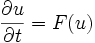$\frac{\partial u}{\partial t} = F(u)$

Here:

•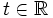$t \in \R$, called the time parameter, and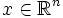$x \in \R^n$, called the space parameter, are the two independent variables
•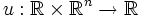$u: \R \times \R^n \to \R$ is the dependent variable, written as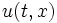$u(t,x)$
•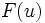$F(u)$ on the right side actually means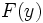$F(y)$ where$y$ is the map sending$x$ to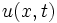$u(x,t)$ (that is,$y$ is the map$u$ at a fixed time)

The solution curves for this (in the space of all possible functions$y$ define the flow of the differential equation) are termed trajectories. By the property of invariance of the differential equation under time-translation, we conclude that the various trajectories through a point are just time-translaets of each other, so there is a unique trajectory curve through each point.

### Definition for a differential manifold

Suppose$F$ is a differential operator on a differential manifold$M$ that is,$F$ takes as input a (sufficiently many times differentiable) function from$M$ to$\R$ and outputs another function from$M$ to$\R$.

The flow equation becomes:$\frac{\partial u}{\partial t} = F(u)$

Here:

•$t \in \R$, called the time parameter, and$x \in M$, called the space parameter, are the two independent variables
•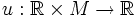$u: \R \times M \to \R$ is the dependent variable, written as$u(t,x)$
•$F(u)$ on the right side actually means$F(y)$ where$y$ is the map sending$x$ to$u(t,x)$ (that is,$y$ is the map$u$ at a fixed time)

The solution curves for this (in the space of all possible functions$y$ define the flow of the differential equation) are termed trajectories. By the property of invariance of the differential equation under time-translation, we conclude that the various trajectories through a point are just time-translaets of each other, so there is a unique trajectory curve through each point.

## Properties of the flow equation

### Invariance under time-translation

The flow equation is autonomous with respect to the time parameter -- the only place where the time parameter occurs is where we differentiate$u$ with respect to time.

### Dependence on linear change in the differential operator

Suppose$F$ is a differential operator, and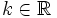$k \in \R$. Then if$u$ is a solution for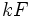$kF$, then the map$(t,x) \mapsto u(kt,x)$ is a solution for$F$.

## Fixed points and trajectories

### Notion of trajectory and local solution

In the case of the flow equation, we are thinking of the thing flowing as a function$y:\R^n \to \R$. Thus, a local solution means a specification of$y$ at all points in some neighbourhood. This means a specification of$u$ for all$x \in M$ and for all$t$ lying inside that time interval.

### Fixed points are solutions of the original equation

A point$y$ is a fixed point of the flow if and only if it solves the original equation$F(y) = 0$. This tells us the following thing:

If the limits of the flow are well-defined at$\infty$ or$-\infty$,, then the limiting points are fixed points of the flow, hence they are solutions for$F$

### Trajectories of the flow

To understand the flow, we can look at the trajectory at each point. Note that by the way the flow is defined, the trajectory is invariant under time-translation of the original differential equation.

The trajectories at a point could be of the following types:

• They could be defined over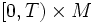$[0,T) \times M$. This phenomenon is called short-time existence of the solution.
• They could be defined over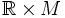$\R \times M$. This phenomenon is called global existence of the solution.

Further, those that satisfy global existence, could satisfy any of the following:

• There exists a limiting point at$\infty$, which is hence a solution to the original differential equation
• Fill this in later

## Groups of diffeomorphisms

### In case of short-time existence

If we have short-time existence at every point, then we get a local one-parameter group of diffeomorphisms acting on the manifold. Namely, we have a local action of$\R$ on$M$, where$t \in \R$ takes$x \in M$ to$u(t,x)$ where$u$ is a solution to the flow equation.

The fact that it is a local one-parameter group of diffeomorphisms follows from the fact that the flow equation in invariant under time translation.

### In case of global existence

If we have global existence at every point, then we get a global one-parameter group of diffeomorphisms viz a global action of$\R$ on$M$ via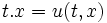$t.x = u(t,x)$. This is a group action because of the invariance under time-translation.

## Supersolutions and subsolutions

### Supersolution to the flow equation

A function$u: \R \times M \to \R$ is termed a supersolution to the flow equation at a point$(t,x)$ if the following holds at that point: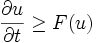$\frac{\partial u}{\partial t} \ge F(u)$

A function is termed a supersolution if it is a supersolution over the entire time range on which it is defined.

### Subsolution to the flow equation

A function$u: \R \times M \to \R$ is termed a subsolution to the flow equation at a point$(t,x)$ if the following holds at that point: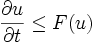$\frac{\partial u}{\partial t} \le F(u)$

A function is termed a subsolution if it is a subsolution over the entire time range on which it is defined.